Clutch Prep is now a part of Pearson
Ch. 17 - Chemical ThermodynamicsWorksheetSee all chapters

# Entropy

See all sections
Sections
Spontaneous Reaction
First Law of Thermodynamics
Entropy
Gibbs Free Energy
Second and Third Laws of Thermodynamics

Entropy is the disorder or chaos associated with a system’s inability to convert thermal energy into mechanical work.

###### Entropy and Spontaneity

Concept #1: The 2nd and 3rd Laws of Thermodynamics

Transcript

Hey guys, in this new video, we're going to take a look at a new variable, the variable of entropy. We're going to see how does entropy relate to the spontaneity of a chemical reaction.
What we're going to say here is, we're going to say that the second law of thermodynamics states that molecular systems tend to move spontaneously to a state of maximum disorder or randomness.
What does this mean? This means that the universe does not want to get ordered. It doesn't want to come together in a nice, little package. The universe naturally wants to go into a chaotic mode. Everything is spinning out of control. Everything is breaking down, becoming less ordered. This is the natural process of our universe.
Simply put, the second law says that the universe is going to shit. Just think of it like that. The universe is getting crappier by the day. This is the natural state of the universe. This is all the second law of thermodynamics is saying.
On the opposite end of this, the third law of thermodynamics says that at absolute zero, absolute zero is zero Kelvin, at absolute zero, the entropy of all objects is equal to zero. So basically everything is always going to be in disorder, always trying to get more and more disordered, unless it's at zero Kelvin. At zero Kelvin, entropy or chaos will stop. Remember, this is the third law of thermodynamics. It goes gains the second law of thermodynamics.
We're going to say that entropy is chaos, disorder, randomness, shittiness. Whatever you want to say it's called. The variable we use for entropy is S.

The 2nd Law of Thermodynamics states systems (chemical reactions) spontaneously move to state of disorder.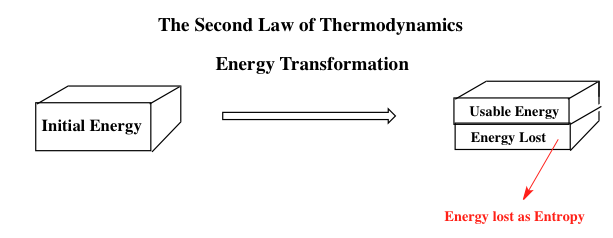Concept #2: Entropy & Phase Changes

Transcript

Now let's think about this I a molecular image-wise. We're going to say, in general, as we move from a solid to a liquid to a gas, what's happening to the molecules of any compound?
Remember, a solid, all the molecules are tightly packed together on top of each other. Then as we become a liquid, the molecules are still around each other, but because it's a liquid, they're naturally sliding over each other, moving around one another. Then as they become a gas, they become very spaced out, so they're bouncing all over the place. We're going to say in this natural progression, when we go from solid, liquid to gas, we're going to say entropy or chaos, will increase because the molecules get farther and farther apart. If entropy is increasing, then the sign of entropy will be positive.
Think about it in the opposite way. In the opposite way, we have gases which are very spaced out. They condense into a liquid. Now the molecules are even closer together. They're not frozen in place yet. We haven't gotten to a solid yet, but they're sliding over one another. Then finally, when we get to a solid, they're frozen in place. In this direction, we're going to say that entropy decreases because order is increasing. If entropy is decreasing, the sign of entropy will be negative.

During a phase change as our molecules grow farther apart then entropy will increase.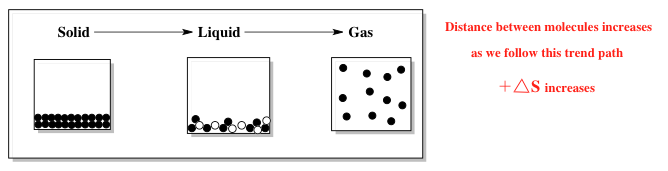During a phase change as our molecules grow closer together then entropy will decrease.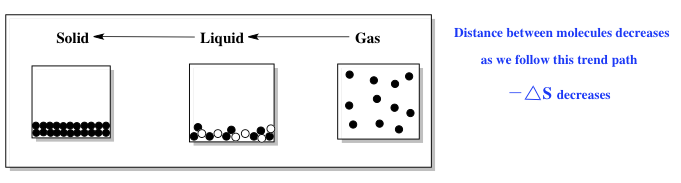Example #1: Which should have the highest molar entropy at 25oC?

Transcript

Now, taking that into consideration, let's look at example one. It says, which should have the highest molar entropy at 25 degrees Celsius. Here, I give you gallium in its three different phases. We have liquid, solid, and gas. Remember, we're just saying who has the highest entropy, we just said who has the most chaos. Who's molecules are most spread out?
This is a very easy question. We're going to say simply the gas phase would have the most entropy because it's the most spread out.

Example #2: Which substance has greater molar entropy.

Transcript

We're going to say which substance, for example two, which substance has greater molar entropy. So this will be – we're going to compare these two, these two and these two. Within those three choices, we're going to choose the best out of each one. The first one we have CH4 gas or CCl4 liquid.
The way you look at these types of questions is you first look at their phases. We first look at phases. All you're going to say here is gases have higher entropy than liquids. And liquids have higher entropies than solids.
If they're tied in the same phase, then we move on to complexity. What the heck do I mean by complexity? All I'm saying is the more elements that make up the compound, the more entropy. The more elements, that make up the compound, then the more entropy.
For example, if I gave you CO2 gas versus S8 gas. You would say that CO2 is made up of 3 different atoms, 1 carbon, 2 oxygens. S8 is made up of 8 atoms of sulfur, so it has more elements that make it up, so it has more entropy. S8 would have more entropy.
If they have the same number of elements that make them up, then we go to overall mass. Here, for three, you're going to say more mass equals more entropy. Because think of it like this, we said that entropy is chaos, disruption, disorder, randomness.
So if we want to think about it, think of it as two cars on a highway, you have a little Mini Cooper versus a Hummer. You're going to say that the Hummer is much larger and if it gets in an accident, it's going to cause more destruction, more disorder. We're going to say the Hummer has a greater mass compared to the smaller car, compared to the Mini Cooper. Just think of it in those terms, more mass equals bigger size equals more chaos that we can have. Here they're different phases, so we can easily say it's the gas.
For the next one, they're both gases, so the first one doesn't help us. Let's look at the second criteria. We're going to say complexity. Here, both have the same number of atoms. One. There's only one neon, one xenon. So that doesn't hep us. Then we move on to the third one, mass. We're going to say that xenon, on our periodic table, has a higher atomic mass than neon. Because of that xenon has a higher entropy.
Then finally, let's look at the last one. We have CH3OH liquid versus C6H5OH liquid. So they both have the same phase again, so the first rule doesn't help us. What we do next is we look at complexity. We're going to say C6H5OH definitely has more elements that make it up, so it's more complex, more moving parts, more chances of chaos and entropy. We're going to say C6H5 has more entropy. You could have also simply said that C6H5 has a greater mass and because of that, it should have the greater entropy.
Remember, delta S is just chaos or disorder. The farther molecules are from each other in their phases, then the greater the entropy. Gaseous compounds have more entropy than liquids or solids. We're going to say that the natural tendency of the universe is to be in disorder.
The second law of thermodynamics is saying that the universe is going to shit or you can just say that the second law of thermodynamics is saying that the entropy of the universe must always be greater than zero because if entropy is always increasing in our universe, there's no chance for it to be less than zero. Always has to be greater than zero. Just remember that for the second law of thermodynamics.

###### Entropy and Phases

When comparing the entropy of different compounds then we must follow a set of guidelines in the following order.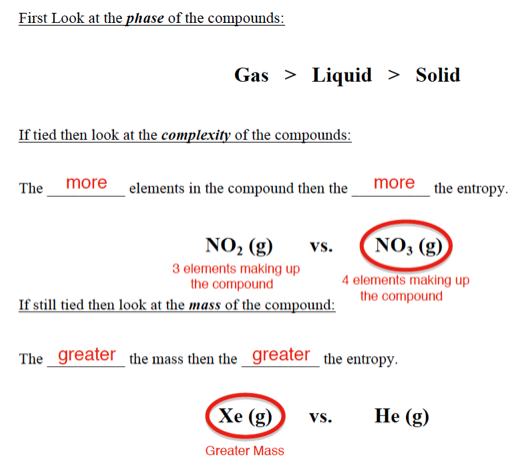Example #3: Arrange the following substances in the order of increasing entropy at 25oC.

Transcript

Hey, guys, in this new video, we're going to continue with our discussion of entropy and the way it relates to spontaneous reactions.
Let's take a look at example one. In example one, it says arrange the following substances in order of increasing entropy at 25 degrees Celsius. So I want in order of increasing entropy, so that means least entropy to most entropy.
Now remember, we talked about the criteria we have to look at to determine who has the most entropy. First, you have to look at the phases. Remember, gases have the most, then liquids, then solids. Now, your professor might be a little crafty in terms of this. Just remember, they might use the word aqueous instead of liquid. We're going to say right now that those words are interchangeable. Liquid is just a solvent that's dissolving a compound. Aqueous means that it's dissolving within our liquid. We're going to basically say they're similar to one another.
Next, if they have the same phase, then we look at complexity. Remember, the more elements that you have within the compound that make it up, the more complex it is and, therefore, the more entropy it has. Let's say for example, we were looking at two compounds that had the same phase. Let's say we were looking at C2H6, ethane gas, and C3H8, propane gas, They're both gases, so then we have to look at complexity. We're going to say propane, which is C3H8, is made up of 11 atoms, 3 carbons, 8 hydrogens. Ethane, on the other hand, is only C2H6. It only has 8 elements making it up. The more elements within your compound, the more complex it is, therefore, the higher its entropy. C3H8 will be higher.
Next, we're going to see if they have the same number of atoms. Then we look at mass. We're going to say the greater the mass, the greater the entropy. Remember the whole analogy, we have two cars out of control on a highway, who's going to cause more damage? The Mini Cooper or the Hummer. The Hummer is much larger. It has greater mass. Therefore, it can cause greater damage.
Let's take a look. To put them in order we have a gas here and we have a gas here. Those two we'd expect to have the highest entropy. Because they're both gases, we go on to the next criteria, complexity. HI is made up of two different elements, H2 is also made up of two elements. So they are tied for complexity. The tie breaker has to be mass. HI has greater mass, so HI should have greater entropy.
Now that we're done with gases, we can move on to aqueous and liquids. Here, we have liquid and liquid. We're going to say Hg is liquid and Br2 is liquid. Br2 has greater complexity because there's two bromines together. Then mercury would be next.
Next, we're going to look at is xenon tetrafluoride, XeF4 versus BaO. They're both solids, right? So they're tied in phase, so we have to look at complexity. The one with more complexity will have greater entropy. Xenon tetrafluoride is XeF4, that's 5 elements that make it up. BaO is only two elements that make it up. It would be xenon tetrafluoride, solid. Then finally, barium oxide would have the least entropy because it's a solid, which has the lowest and also it's only made up of two elements, therefore, it's complexity is much longer than XeF4.
Hopefully, you guys were able to follow along with these three criteria. Just remember, look at phase first, then look at complexity. If that all fails, look at mass. The greater the mass, the greater the entropy.
Now that we've seen this example, let's move on to example two.

Example #4: Containers A and B have two different gases that are allowed to enter Container C. Based on the image of Container C, what is the sign of entropy, ΔS°.

Transcript

Example two says we have container A, which is this one, and container B. They have two different gases. We allow both of these gases to funnel into a new container, container C. Just to let you know, it's not drawn to scale. All these gases in here, are in here. Even though I don't show all of them, consider all of them to be in there. All the gases here are also in here.
Based on the image of container C, what is the sign of entropy, delta S? Here this little zero here just means standard conditions. When we say standard conditions, we mean 1 atmosphere and 25 degrees Celsius. That's our standard-state when we're talking about thermodynamics.
Don't get confused. We also dealt with standard conditions when we deal with gases. We say STP, now STP for gases is different. Under those standard conditions, the pressure is still 1 atmosphere, but the temperature is 0 degrees Celsius. When we're talking about spontaneity and thermodynamics, the new temperature now becomes 25 degrees Celsius.
Just think about it like this, delta S is chaos. The more disorder, the better. We have one container filled with gases. We have a second container filled with gases. We take those two containers, which are very chaotic, and dump them all together into an even bigger container where they can just mix around even more, bouncing all over the place.
Ask yourself, if I take both gases and I put them together, will the entropy increase or decrease. I have a party of 100 people. I have another party of 100 people. I take them and now I have a party of 200 people. Will they get even crazier together than apart? That's how you have to think of it. We're going to say here, the more gases you put in, the crazier things are going to get because they're going to be bouncing around the wall because there are even more of them. We're going to say here entropy is positive. We should get a positive entropy when we mix gases together because we're causing greater disruption, greater chaos.
Now that we've done examples one and examples two, I want you guys to at least attempt to do this following practice question. I'll give you guys a few hints. Here we're saying an ideal gas is allowed to expand at constant temperature. What are the signs for delta H, delta S and delta G?
First of all, for delta S, remember, S is chaos, entropy. Here the gases in A are becoming like the gases in B. They transition and now they become like the picture in B. Ask yourself, since the gases have more room to bounce around, will their entropy increase or decrease.
Next, delta G. I'll tell you this, delta G when it's negative, it's spontaneous. When it's positive, it's non-spontaneous. Ask yourself this, an ideal gas is a gas that wants enough room for itself. Based on that information is delta G positive or negative.
Finally, delta H is enthalpy. Enthalpy is just the measurement of a reaction if it absorbs energy or if it releases energy. When we talk about energy, we're talking about heat energy in this case. If it's absorbing energy, it's going to be positive. If it's releasing energy, it's going to be negative. I say constant temperature. What's the sign of delta H when we realize that we're at constant temperature?
These little hints will help you figure out what the answer is. Don't worry, if you can't figure it out, just come back and click on the explanation button and you'll see a video of me explaining how to approach this problem. Good luck guys.

Practice: An ideal gas is allowed to expand at constant temperature. What are the signs of ∆H, ∆S & ∆G.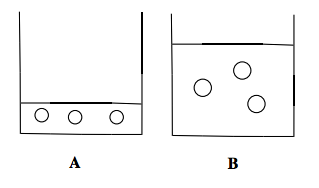Whenever a phase change occurs, first determine if bonds are broken or formed to figure out the signs of enthalpy and entropy. Afterwards, determine if the reaction is spontaneous to determine the sign of Gibbs Free energy.

Example #5: Consider the spontaneous fusion of ice at room temperature. For this process what are the signs for ΔH, ΔS and ΔG?

Transcript

Hey, guys, in this new video, we're going to continue with our discussion of entropy and spontaneous reactions, but now I'll introduce two new variables. Enthalpy, delta H, or Gibbs free energy, delta G.
Let's take a look at this example, it says consider the spontaneous fusion of ice at room temperature. For this process, what are the signs for delta H, delta S, and delta G. Before we even take a look at this question, I'm going to give you guys some notes to help you realize what the sign of delta H and delta S can be.
We're going to say here, if we're going from gas to liquid to solid, what's happening to our molecules? Our molecules are coming closer and closer together until they reach the solid state in which they're frozen in place. We're going to say technically here, we're forming bonds. We're forming intermolecular bonds. Molecule A is coming closer to molecule B, so they're forming an attraction to each other. It's not a real bond, it's more of an intermolecular force bond. We're going to say here if you're forming bonds, then we must be releasing energy in order to form those bonds. Delta H would be negative.
On the opposite end, we're going to say delta S, what's happening to the entropy? We're becoming more ordered. Bonds are coming closer and closer together, now you're a solid, so you're entropy should also be negative.
Now if we go the opposite way, we go from solid to liquid to gas, what's happening? We're breaking these intermolecular bonds. Remember, to break bonds, you have to first absorb energy or heat. You use that energy you just absorbed in order to break those bonds. If you're absorbing energy, your delta H will be positive.
At the same time, our delta S, what's happening to the molecules? They're becoming more and more like gases. Gases have tons of chaos. Chaos is increasing, so delta S should be positive.
This is the way you have to understand it, whether you're forming bonds or breaking bonds, will help you figure out are you having a delta H and delta S that's positive or are they negative.
If we go back to the question, it says fusion. Now, the name can be a little bit deceiving. Scientifically, fusion means melting. When it comes to phase changes, fusion just means melting. If you're melting, what phases are you going between? Remember, what melts? We're going to say if you go from a solid to a liquid, you're melting. If you're going from a solid to a liquid, what are you doing to the bonds? You're breaking them. Therefore, delta H and delta S should both be positive. Because of that, that takes out B, C, and E. Our answer is either A or D in this question.
Now, the next important piece of information I give to you is the word spontaneous. Because I say it's spontaneous, remember we've said this earlier, if it's a spontaneous reaction, delta G has to be negative. If it's spontaneous, delta G is negative. That one word that I use, makes it answer choice D.
Just remember, are you forming bonds or breaking bonds? This will help you figure out delta H or delta S. For delta G, you have to think in your head. Does this naturally occur? Does it make sense for this to occur? If it does, then it's a natural process. If it's a natural process, it's spontaneous. If it's spontaneous, delta G, will be negative.
Knowing this I want you guys to attempt to do the next question on your own. Here we say consider the freezing of liquid water at 30 degrees Celsius. Just remember, does freezing mean you're making or breaking bonds. This will help you figure out what delta H and delta S are in terms of signs.
Then ask yourself this question freezing of water at 30 degrees Celsius, is that a spontaneous reaction, which is natural, or is it non-spontaneous. If it's spontaneous, delta G will be negative. If it's non-spontaneous, delta G will be positive.
Knowing all this, try to answer this question as best as you can. Once you're done, click on the explanation button and take look at a video of me explaining how to approach this problem. Good luck guys.

Practice: Consider the freezing of liquid water at 30°C. For this process what are the signs for ∆H, ∆S, and ∆G?

If bonds are broken then the entropy of a reaction increases, but if bonds are formed then the entropy of a reaction decreases.

Practice: Predict the sign of ∆S in the system for each of the following processes:

a) Ag+ (aq) + Br - (aq) → AgBr (s)

b) CI2 (g) → 2 CI - (g)

c) CaCO3 (s) → CaO (s) + CO2 (g)

d) Pb (s) at 50°C → Pb (s) at 70°C

Practice: For each of the following reactions state the signs of ∆H (enthalpy) and ∆S (entropy):

a) Fusion of ice.

b) Sublimation of CO2

c) Vaporization of aqueous water.

d) Deposition of chlorine gas.

e) Condensation of water vapor.

###### Entropy and Calculations

The 2nd Law of Thermodynamics states that the entropy of the universe is always increasing and so it must always be greater than zero.

Concept #3: The Universe & Entropy

Transcript

Hey, guys, in this new video, we're going to take a look at calculating entropy for a system.
First of all, before we begin, just remember, we have the term, system. When we say the word system, we mean that this is the portion of the universe that we're concerned with. When we say system, we're really talking about the balanced chemical equation. A system is just our balanced equation that we're looking at.
We're saying that the second law of thermodynamics talks in terms of the system and it says that the entropy of a system increases spontaneously. Remember, the entropy of the universe is gradually increasing. The world is getting more and more chaotic.
Now, we're going to say that we can't only focus on our system. Besides our system, we have our surroundings. We're going to say our systems plus our surroundings gives us our entropy total or our universe. System plus surroundings gives us our total universe.
Now we're going to say thus to calculate the total entropy change, delta S total, we use the following equation. We're just going to say delta S total is the same thing as delta S universe and that just equals delta S of my system plus delta S of my surroundings. Remember, when we say system, we really mean reaction. Delta S system could also mean delta S of our reaction.

The entropy of the universe takes the look at the entropy of our system (the chemical reaction) and of the universe.

Concept #4: Total Entropy & Spontaneity

Transcript

Now we're going to say here in terms of spontaneity, we're going to say if our delta S total is greater than zero, then the reaction is spontaneous. If it's less than zero, then it's non-spontaneous. If it's equal to zero, then our reaction is simply at equilibrium.
Just remember that, we have to take into consideration both our system and our surroundings as a whole to determine if our reaction is spontaneous overall or not because sometimes your delta S system may be highly spontaneous, meaning that its delta S value is very positive, but if we add in the surroundings and at the end we get a total that's negative, then overall, it would be non-spontaneous. Have to take into consideration both aspects, our system and our surroundings.

If the entropy is greater than zero then we classify the process as spontaneous.

Concept #5: The Entropy of a Reaction

Transcript

If we take a look here it says calculate the standard entropy in kilojoules of a reaction at 25 degrees Celsius for the following reaction, so we have one mole of nitrogen gas combining with three moles of hydrogen gas to give us two moles of ammonia gas as product. Here I give you the standard molar entropies of N2, H2, and NH3 as these numbers respectively.
Any time your professor asks you to find the delta S of our system, they're really saying the delta S of our reaction. That is just products, the summation of products, minus the summation of reactants. Products minus reactants. Remember, this also goes for delta G and delta H. Sometimes you'll be asked to calculate the standard enthalpy, delta H, or the standard Gibbs free energy, delta G. In those cases, delta G system, delta H system are products minus reactants.
Here we're just going to say it equals – we have, remember, the coefficients, 2, 3, and 1. We're going to say according to our balanced equation, we have 2 moles of NH3. Each NH3 is this total right here. That's our products minus our reactants. We have 1 mole N2. Each one is 191.5 joules over K times moles plus the other reactant, which is 3 moles of H2 times 130.6 joules over K times moles.
Now, we can see that the moles will cancel out. Our units here will be in joules over Kelvin. When we multiply the 2 times the 192.3, that's going to give us 384.6 joules over Kelvin minus everything inside of our reactants boxes. That comes out to 583.3 joules over Kelvin. Now just subtract those two. That gives me negative 198.7 joules over Kelvin.
Remember, I asked for the answer in kilojoules, not in joules, so we have to do one more conversion. We want kilojoules. One kilo is 10 to the 3 joules. Remember 10 to the 3 is the same thing as 1,000. Joules cancel out. Our units at the end will be kilojoules over Kelvin. It's going to be negative 0.1987 kilojoules over Kelvin. That will be our answer.
Just remember if they ask you to find the standard entropy of our reaction, it's just products minus reactants.
Now, one more thing we need to realize here, just before we move on to the next set of videos. Just remember, here we're going to say that N2 and H2 are both in their natural states. Remember your natural state means you're by yourself, you're an element by yourself. Example, carbon graphite or sodium solid or you're connected to copies of yourself, such as N2 or H2 or S8 or P4. If you're by yourself, you're connected to copies of yourself, that's your natural state.
We're going to say here although N2 and H2 are both in their natural states, their delta S values are not equal to zero. Remember delta S value is only equal to zero if the temperature is at absolute zero, zero Kelvin.
We're going to say that for an element in its natural state, delta H and delta G both equal zero. If they gave us the values for N2 and H2 here, they both would be zero when we're looking at the delta H and their delta G values. Why? Because they're in their natural states. I just want you guys to remember this. For something in its natural state, it means it's by itself or it's connected to copies of itself. In either case, delta G and delta H equals zero.
Delta S can only equal zero for those compounds if we're at zero Kelvin. Remember that's the third law of thermodynamics. Just remember that difference and you guys can answer those types of theory questions when you see them on exams.

The entropy, enthalpy and Gibbs Free energy of a reaction is equal to products minus reactants.

Example #6: The oxidation of iron metal is given by the following reaction:

Practice: Diethyl ether (C4H10O2, MW = 90.1 g/mol) has a boiling point of 35.6oC and heat of vaporization of 26.7 kJ/mol. What is the change in entropy (in kJ/K) when 3.2 g of diethyl ether at 35.6oC vaporizes at its boiling point?Concept #6: Trouton's Rule & Boltzmann's Equation

Transcript

Hey guys. We're not going to continue with our discussion on solving questions that deal with spontaneity and entropy. So, let's take a look at this example, it says, the normal boiling point of liquid propane is 231 Kelvin, what is the enthalpy of vaporization of liquid propane. Now, first of all. Remember, they don't have to tell you explicitly enthalpy of vaporization, instead of saying enthalpy they could have said heat of vaporization, in either case it just means Delta h. So, all they're telling me here is the normal boiling point of liquid propane and from that I'm supposed to figure out what my Delta h is.